# Calculating Military Time Intervals in Microsoft Excel 2010

In this article, we will learn how to calculate military time intervals. We will use the “Text” and “DOLLARDE” functions in Microsoft Excel 2010.

TEXT: -This function is used to convert a value to text in a specific number format.

Syntax of “TEXT” function:    =TEXT (Value, format_text)

For Example: -

• Cell B2 contains the date 12-Feb-2013
• Write the formula in cell C2 =TEXT(A2,"MMMM"), and press Enter on your keyboard.
• The function will return the month name.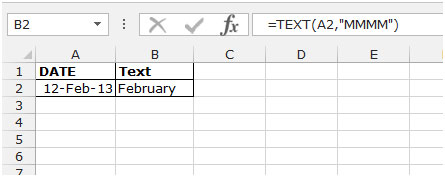DOLLARDE: -This function is used to convert a dollar price, which is expressed as a fraction, into a dollar price reflecting as a decimal number.
Syntax of “DOLLARDE” function:    =DOLLARDE (fractional_dollar, fraction)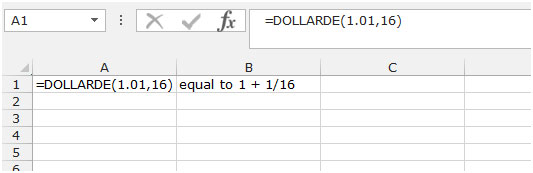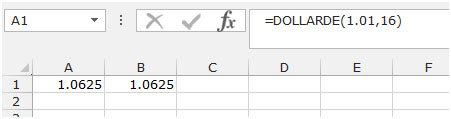Let’s take an example to understand how we can calculate military time intervals.

We have data in the range A1:B10. Both the columns contain the interval timings, and formatted in military style (hhmm). Now, we want to return the interval timings.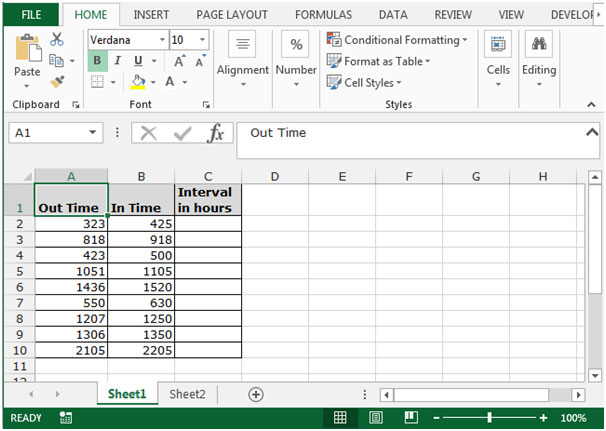To find the interval in between pairs of times when they are formatted in Military style (hhmm), follow below given formula:-

• Write the formula in cell C2.
• =(TEXT(B2,"00\:00")-TEXT(A2,"00\:00"))*24
• The function will return the break timing in between the pairs of times.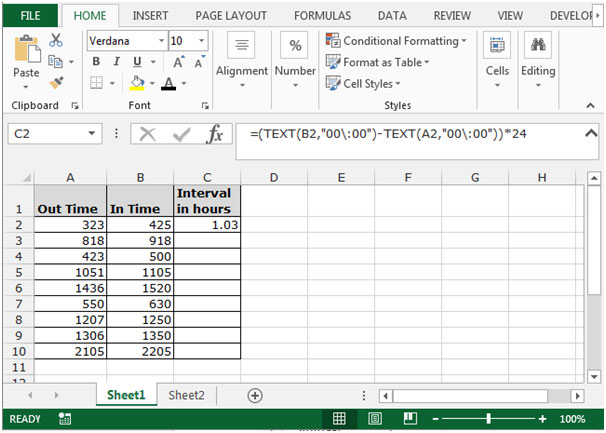• Copy the same formula by pressing the key Ctrl+C and paste in the range C3:C10 by pressing the key Ctrl+V on your keyboard.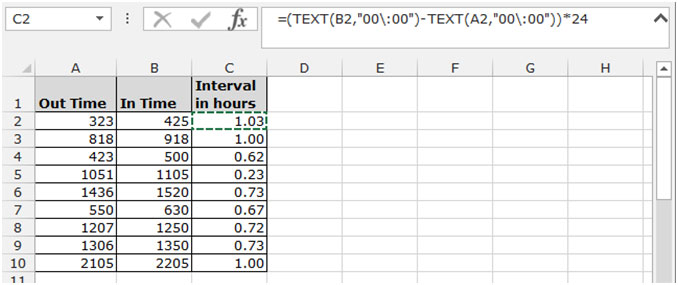There is another formula to return the same result. To apply that formula, follow below given steps:-

• Write the formula in cell C2.
• =DOLLARDE(B2/100,60)-DOLLARDE(A2/100,60)
• The function will return the break timing in between the pairs of times.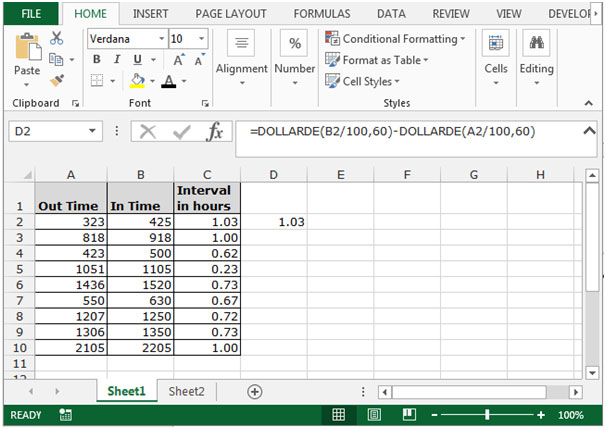These are the ways by which we can calculate the interval timings from In and Out timings which are formatted as military timings.

## Users are saying about us...

1.This is very useful but what to do if the list of time contains start time: 2300, end time: 0715?? because I'm getting a negative result.

•Using the TEXT function from the example above, this IF function worked for me:

=IF(B2<A2,(TEXT(2400+B2,"00\:00")-TEXT(A2,"00\:00"))*24,(TEXT(B2,"00\:00")-TEXT(A2,"00\:00"))*24)

- or, this works as well -

=IF(B2<A2,(1+TEXT(B2,"00\:00")-TEXT(A2,"00\:00"))*24,(TEXT(B2,"00\:00")-TEXT(A2,"00\:00"))*24)

Terms and Conditions of use

The applications/code on this site are distributed as is and without warranties or liability. In no event shall the owner of the copyrights, or the authors of the applications/code be liable for any loss of profit, any problems or any damage resulting from the use or evaluation of the applications/code.